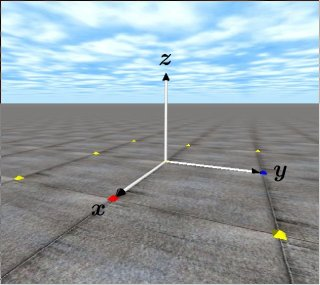# ODE Tutorial 11: Position and OrientationThe coordinates system of ODE (right hand system)

ODE (Open Dynamcs Engine) lecture time it is 11th. This time the method where the system of coordinates of ODE and position attitude of rigid body it sets and acquires and is explained.

System of coordinates

The system of coordinates of ODE as shown in the upper figure, is orthogonal coordinate of the right hand type which is used generally in physics and mathematics. In the center of the small pyramid where 9 there is a starting point, red pyramid direction x axis, blue pyramid direction the Y-axis and sky direction has become the z axis from the center. Furthermore, being to be good with anything, it does, system of units, but because with this lecture SI system of units is adopted, each pyramid has lined up at the interval at a time of 1m.

Setting of position and orientation

In order to set rigid body with respect to 3 dimensional spaces, it is necessary to appoint position and attitude. As for attitude it is possible to decide with rotational transform queue, by API below it is setting possible even with ODE.

void dBodySetPosition (dBodyID, dReal x, dReal y, dReal z);
Center of gravity of rigid body dBodyID is set position of world coordinate system (x, y, z).

void dRFromAxisAndAngle (dMatrix3 R, dReal ax, dReal ay, dReal az, dReal angle);
When axis of rotation vector (ax, ay, az) the around angle [ rad ] turning in anticlockwise, rotation matrix R is acquired.

void dBodySetRotation (dBodyID, const dMatrix3 R);
Attitude of rigid body dBodyID is set to revolution queue R.

In addition, with ODE API and void dBodySetQuaternion in addition regarding quaternion (dBodyID and const dQuaternion q) it is.

Acquisition of Position and Orientation

Const dReal * dBodyGetPosition (dBodyID);
Position of rigid body dBodyID is acquired. As for return value the pointer to the arrangement to which position with world coordinate system is housed.

Const dReal * dBodyGetRotation (dBodyID);
Revolution it forms a line acquires of rigid body dBodyID. As for return value the pointer to the arrangement to which revolution queue is housed.

A Sample Code
The next sample cord/code position (0.0, 0.0, 1.0), 45 degrees (M_PI/4.0) has set the cylinder to the attitude which turned around x axis. Furthermore, the function printPos which indicates the balance station of the object () it added. Furthermore, the cylinder unless attitude is set, has become attitude of standing upright where the extended shaft matches z axial direction.

[code]
typedef struct {
dBodyID body;
dGeomID geom;
} MyObject;
MyObject pillar;

void createPillar()
{
dMass m1;
dReal radius = 0.1, length = 0.5, mass = 1.0;

pillar.body = dBodyCreate(world);
dMassSetZero(&m1);
dBodySetMass(pillar.body,&m1);
dBodySetPosition(pillar.body,　0.0, 0.0, 1.0); // Set a center of gravity to (0.0, 0.0, 1.0)
dMatrix3 R;
dRFromAxisAndAngle(R, 1.0, 0.0, 0.0, M_PI/ 4.0);　// rotatio π/4[rad] around the x axis
dBodySetRotation(pillar.body,R);
dGeomSetBody(pillar.geom,pillar.body);
}

void printPos(dBodyID obj_body) // print a position of COG
{
const dReal *pos;
dReal x, y, z;
pos = dBodyGetPosition(obj_body);
x = pos;
y = pos;
z = pos;
printf(“x=%f y=%f z=%f \n”, x, y, z);
}

[/code]

To be continue.

english
スポンサーリンク
demura.netをフォローするdemura.net

タイトルとURLをコピーしました